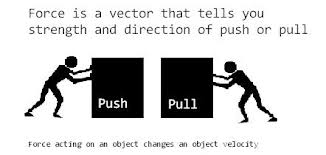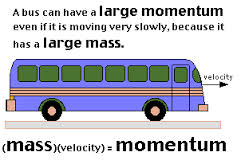# Difference between Force and Momentum

##### Key Difference: Force is defined as any pull or push which is exerted on a body due to the body’s interaction with another object or body. Momentum is basically used to measure the quantity of motion of an object. It can be described as the property of a moving object to keep moving in the same direction, and thus it makes it difficult to stop.Force is defined as any pull or push which is exerted on a body due to the body’s interaction with another object or body. Due to this push or pull the object starts moving or stops moving. One can simply find an example of force by looking at a person kicking a football. The force exerted on the football due to the foot is responsible for the movement of the ball.

A force may be responsible for causing an object to – speed down, speed up, change in direction or change in shape.

Three features that are concerned with the force are –

The magnitude of the force

The direction in which the force is applied

And the point at which the force is being applied

Newton is the SI unit of force, and it is measured with a newton meter.Momentum is basically used to measure the quantity of motion of an object. It can be described as the property of a moving object to keep moving in the same direction, and thus it makes it difficult to stop. Momentum of the body is just equal to the body’s mass multiplied by its velocity. It is measured in Ns.

A light car and a heavy car are travelling at the same speed, but still stopping a heavy car would require more efforts than stopping a lighter car. This is due to the momentum of the heavy car which is greater than that of the lighter car. The direction of momentum is the same that of velocity. It is a vector quantity. The SI unit for momentum is kg m/s.

Force and momentum are related to each other. Force acting on an object also changes its momentum. Force can also be described as the time rate of change in momentum. Force is the phenomenon between two objects. On the other hand, momentum is within an object.

Comparison between Force and Momentum:

 Force Momentum Definition Force is defined as any pull or push which is exerted on a body due to the body’s interaction with another object or body. Momentum is basically used to measure the quantity of motion of an object. It can be described as the tendency of an object to keep moving in the same direction. Unit of measurement The unit for force is the Newton which is abbreviated N. The unit for momentum is kg m/s. Types Some types of forces are - Gravitational – attractive force which is exerted between bodies due to their masses Air resistance or drag – air resistance is the force which is encountered in air opposing the movement of objects. Drag is generally used for the opposition force in any gas or liquid. Normal Reaction or contact – it refers to the repulsive force which prevent the two touch bodies to move into each other Friction – it opposes motion Upthrust – it refers to the force which is felt by the objects wen they are place into a fluid. Angular Momentum – it is the product of body’s mass and its angular velocity Linear Momentum – it is the product - this momentum is related to the linear motion of the object Formula Net Force = mass * acceleration Momentum = mass * velocity Properties It is a vector quantity Direction of the force depends upon the direction from which it is coming, and it remain constant until it gets changed by some other force It is a vector quantity It has same direction as velocity It helps in understanding collisions

Image Courtesy: physics.tutorvista.com, batesville.k12.in.us